﻿ Investigations of Dynamic Characteristics of Eccentric Rotary Shaft of Wankelengine

### Investigations of Dynamic Characteristics of Eccentric Rotary Shaft of Wankelengine

N. D. Pagar, S. H. GawandeOPEN ACCESSPEER-REVIEWED

## Investigations of Dynamic Characteristics of Eccentric Rotary Shaft of Wankelengine

N. D. Pagar1,, S. H. Gawande2

1Department of Mechanical Engineering, RMD Singhad School of Engineering, Pune, India

2Department of Mechanical Engineering, M. E. Society’s College of Engineering, Pune, India

### Abstract

Accurate prediction of critical speeds in rotating machinery is of great importance to designer and many attempts have been made to calculate it exactly. At the design stage it is necessary to predict accurately the dynamic behavior of rotating system of rotating parts of Wankelengine in order to avoid resonant conditions at operating speeds. Critical speed of a rotating shaft differs from its non-rotating natural frequency. The main reason for this difference is known to be the gyroscopic momentum. So it is quite great important to determine the natural frequency of the eccentric shaft in non-rotating condition (free-free condition) i.e. degrees of freedom are not restricted. In this study the natural frequency and mode shapes are predicted for the eccentric shaft in free-free condition (non-rotating) by using the commercial software package (ANSYS) in its modal analysis option. And results obtained from it are compared with experimental modal analysis (FFT analyzer). The verified results leads to the prediction of the dynamic behavior of the eccentric shaft viz. design calculations, natural frequencies, mode shapes.

### At a glance: Figures

123
Prev Next

• Pagar, N. D., and S. H. Gawande. "Investigations of Dynamic Characteristics of Eccentric Rotary Shaft of Wankelengine." Journal of Mechanical Design and Vibration 2.2 (2014): 53-59.
• Pagar, N. D. , & Gawande, S. H. (2014). Investigations of Dynamic Characteristics of Eccentric Rotary Shaft of Wankelengine. Journal of Mechanical Design and Vibration, 2(2), 53-59.
• Pagar, N. D., and S. H. Gawande. "Investigations of Dynamic Characteristics of Eccentric Rotary Shaft of Wankelengine." Journal of Mechanical Design and Vibration 2, no. 2 (2014): 53-59.

 Import into BibTeX Import into EndNote Import into RefMan Import into RefWorks

### 1. Introduction

The demands on a modern engine system are increasing rapidly. High performance engines, low fuel consumption, low noise and vibrationetc are the several factor a consumer demands simultaneously. These demands pressurize engineers to upgrade engine designs and require optimization of engine components. Since the eccentric shaft is one of the key component in the rotary engine system. Identification of dynamic behavior plays an important role in the design, thus an accurate prediction for the dynamic characteristics of the system using finite element method is essential for modern equipment .

As the speed of rotation of a machine is radially increased, it will be observed that at certain speeds the shaft may vibrate violently whereas at speeds below and above of these it will run relatively quietly. The speeds at which the several vibrations occur are known as the critical speeds of the shaft. If unit operates at or near a critical speed, large amplitudes will be built up. Such a condition result in dangerously high stresses, possible rubbing of the rotating parts, and an undesirable vibration being transmitted to the foundations. It is therefore quite important that the shaft should designed so that the critical speeds are 20 to 30% above or below the running speed also the determination of the fundamental natural frequency is very important in its torsional and flexural mode .

1.1. Phenomenon Description

Consider a cylindrical shaft with inner radius ‘a’, outer radius ‘b’ and length ‘L’, which rotates with a constant rotational speed ‘ω’ as shown in Figure 1. The shaft is supported on two bearings at shaft ends, which suppress axial movement of the shaft.

Shaft rotation produces a centrifugal force that creates a radial stress in the shaft. It is well known that because of the Poisson effect, normal stresses in one direction produce normal strain in lateral direction. If the strain in the lateral direction is forced to zero, a normal stress in that direction will be created in order to compensate the strain. Similarly, the radial stress in the rotating shaft creates axial stress in the shaft. The sum of the axial stresses on the cross section of the shaft produces net axial forces in it. The existence of axial force changes the natural frequency of lateral frequency of lateral vibration of the shaft. Hence, in this manner shaft rotation can change its natural frequencies .

1.2 Action of Critical Speed

The eccentric shaft carries different mountings; critical speed of a rotating shaft differs from its non-rotating natural frequency . So the fundamental natural frequency determination in the non-rotating shaft is important. The Figure 2 shows the variation of critical speed with fundamental natural frequencies which is always increases.

The centre of gravity of these mounting does not coincide with that of the shaft and lies a little away from the shaft axis. This will force the shaft to bend. When the shaft starts to rotate with the mountings, a centrifugal force is developed which increases with the speed, which further increases the centre of gravity from the axis of rotation. This effect is cumulative and at a particular speed, the shaft fails. This speed at which the deflection of shaft from the axis of rotation becomes infinite is referred to as the critical speed of the shaft. This is dangerous phenomenon and it is highly imperative to calculate the critical speed so as to operate the engine at safe speeds .

As per  Kimball and Hullexplain the physical action which takes place while the shaft passes through the critical speed. They show that below the critical speed the centre of mass rotates in a circle about the geometric centre, whereas above it the shaft rotates about the centre of mass. Thus the axis of rotation is changed at critical speed from geometric centre to mass centre. When the shaft rotates at the critical, the restoring force of the shaft is neutralized and the action is dynamically unstable, hence large amplitudes of vibration may occur .

The critical speed coincides with the natural frequency of vibration of the shaft, which may be found by the principle developed by Lord Rayleigh . Lord Rayleigh found that a body vibrating at its natural frequency does so with simple harmonic motion and that all points on the body come to rest at same point and attain their maximum velocity simultaneously, even though they are completely out of phase.

In this study, the non-rotating mode shapes, natural frequencies of eccentric shaft are used in a modal analysis method for calculating the dynamic characteristics. Nowadays, modal analysis has become a useful tool for studying the dynamical behavior of complex mechanical structures . However, the analysis of extensive data sets that are obtained during high channel-count modal testing requires additional efforts for the development of dedicated modal parameter estimation methods. The results obtained from the natural frequency and mode shapes are computed by the compatible FEA software like ANSYS. And it leads to the verification for the experimental results. The approach of the software validation results is more convergent to the experimental results but the conditions in an FE model should be as close to reality as possible to ensure acceptable accuracy. It is normal, however, to assume uniform initial conditions, such as thickness distribution and yield strength, in all parts in mechanical simulations. Often that is reasonable assumption, but there are several examples of instance where non-uniform properties caused by the manufacturing processes produce significant effects on the product performance.

### 2. Modal Analysis

In operation, all machines and their supporting structures, vehicles and building are subjected to dynamic forces which cause vibrations. Very often the vibrations have to be investigated, either because they can cause immediate problem, or because the structure has to be cleared to a “standard” or test specification. Whatever the reason, we need to quantify the structural response in some way, so that its implication on factors such as performance and fatigue can be evaluated.

2.1. Modal Frequency Response

The equation of motion for a total system with harmonic excitation may be determined as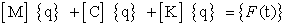(1)

For harmonic excitations expressed in terms of frequencies, {F(t)} = {ƒ(ω)eiωt}, and for harmonic motion, a harmonic solution in terms of the modal coordinate {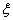(ω)} is assumed.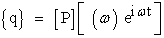(2)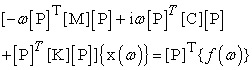(3)

Where;

[P]T [M][P] = generalized mass matrix

[P]T [K][P] =generalized stiffness matrix

[P]T [C][P]= generalized damping matrix

[P]T {ƒ (ω)} = modal force vector

For the case of modal damping, each mode has damping Ci, where Ci = 2miωii. The equation remains uncoupled and has the following form for each mode and mode responses are computed as;(4)

Each of the model responses is computed by using this.

Historically, in order to avoid complexities of dynamic analysis, engineers usually have designed excessively bulky and massive structures. Dynamic considerations were not at all taken into account while designing the components. But these massive structures did not solved all the problems and were susceptible to failures arising out of excessive vibrations since increase in mass is always accompanied by decrease in natural frequencies of the structure. Nowadays, high energy costs and more stringent consumer demands for performance and safety have necessitated the development of structural components that cost less, last longer and are less expensive to operate. At the same time, these products must carry greater loads, run at faster speeds with lower noise, vibrate less and fail less frequently. Such tough design constraints no longer allow dynamics problems to be tackled by using traditional “rule of thumb” approaches .

Today’s engineer must have deeper insight into how a structure will respond in its operating environment. To predict structural strength and reliability, it very much important to determine the dynamic deflections, loads and stresses. Dynamic analysis permits to predict more user-oriented criteria such as comfort and noise.

Thus, by understanding the dynamics of a structure through analysis, optimum designs can be developed from a rational data base. Experimental Modal testing plays an important role at this stage of design. It enables us to understand the dynamic characteristics of the structure which can be effectively used in the design of structures from dynamics point of view. The dormant potential of this technique is being widely implemented nowadays in aerospace industries, nuclear reactor designs, automobile industries, etc.

Experimental modal testing is the process of characterizing the dynamic properties of an elastic structure by identifying its modes of vibration. That is, each mode has a specific natural frequency and damping factor, which can be identified from practically any point on the structure. In addition it has a characteristic “Mode shape” which defines the resonance especially over the entire structure. The above three parameters, viz. Modal frequency, modal damping and mode shape called as “Modal Parameters” constitute a complete description of the structure within the frequency range of interest .

2.2. Modal Model

The first step in dynamic analysis is to define the dynamic properties of the structure. This is done by specifying either;

The mass, damping and stiffness distribution, or

The modes of vibration of the structure.

Based on a structure’s material properties, geometry and boundary conditions, an analytical model can be formulated which describes structural dynamic properties in terms of mass, damping and stiffness distribution. Equations of motion developed from the model are solved to determine the structure’s response to any externally applied forces. Because these equations contain the response motions of the structure as functions of time, the description of its mass, damping and stiffness distribution is called a Time Domain Model.

An alternative model of the structure, which describes its dynamic properties as a function of frequency, can be developed. This involves defining each of the structure’s component modes of vibration. Each mode is defined by three modal parameters;

1. A undamped natural frequency

2. A measure of energy dissipation i.e. damping

3. A characteristic deflection shape i.e. mode shape.

The natural frequency, damping and mode shape for each of the structure’s modes of vibration comprises its Modal Model. The Modal Model and the Time Domain Model are equivalent descriptions of a structure’s dynamic properties. Basically there are two techniques whereby the modal model of a structure can be obtained viz.

• Mathematical modeling ( Analytical methods )

• Modal Testing

With a view to comparing the methods used to obtain a modal model, it is thought fit to discuss them briefly as follows: -

Mathematical Modelling:

The development of mathematical model can be done by two methods;

1). Lumped Parameter Systems

2). Finite Element Method

a) Lumped Parameter Systems:

Mathematical models describe a structure as a distribution of mass, stiffness and damping. Although all structures are continuous, in some cases, their dynamics can be represented adequately by assuming that the structure behave as an assemblage of rigid masses connected together by spring and damper elements, Equations of motion for these lumped parameter systems are easily derived by applying Newton’s second law.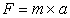(5)

This will yield one equation for each mass in the system. Combined, they are called the structure’s Equations of Motion and can be written in matrix form as: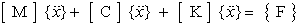(6)

where, all the matrices have their usual meaning. The above equations can be solved for finding out the eigenvalues and eigenvectors i.e. the natural frequencies and mode shapes, by a number of methods. Thus, the modal properties of the structure can be determined using lumped parameter method.

b) Finite Element Method:

Many dynamics problems cannot be solved by lumped parameter models as a more accurate modeling of the distributed elastic behavior of the structure is needed. Structures with complex geometry, material properties or boundary conditions, are modeled using numerical methods that provide approximate but acceptable solutions.

The FEM is one of the most powerful and popular mathematical modeling techniques. In modal analysis we are basically interested in finding out eigenvalues (natural frequencies) and eigenvectors (mode shapes).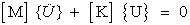(7)

Here as the nodal displacements are time varying the displacement vector {} can be represented by assuming a simple harmonic motion with angular frequency of motion (rad / sec.). As,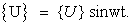(8)

Substituting eq.  in eq. ,(9)

We can write the above equation in general form as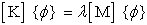(10)

where,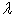= constant= mode shape vector

If the solution for say ‘p’ eigenvalues and eigenvectors are sought, the eq.  can be rewritten as;(11)

Where {} is a (n × p) matrix with its column equal to ‘p’ eigenvectors andis a p×p diagonal matrix listing the corresponding eigenvalues, Standard finite element procedure is used to find out the stiffness and mass matrices in the above equations. The solution of above equations involves solving an eigenvalues/ eigenvector problem. The eigenvalues will determine natural frequencies while eigenvectors give us the mode shapes corresponding to each mode .

Effective computer procedures are available to finally get eigenvalues and eigenvectors from these equations. Thus it can be said that FEM is a refinement of lumped parameters method which improves the accuracy of results.

### 3. Modal Analysis by Finite Element Analysis

ANSYS10.0 is one of the most powerful engineering design and analysis software. ANSYS is a finite element method based package used to analyze the finite element method (FEM) problems. The models can be imported from other modeling software or can be created within ANSYS. In this work model is imported from solid works.

3.1. Geometric Model for FEA

To create the geometric model of eccentric shaft, initially using 2D model and can be extruded in axial direction to obtain the 3D model since the variation of stepped geometry is taken into accountas shown in Figure 3. Geometry is crated into solid works and imported into ANSYS as an IGES (Initial Graphic Exchange Specification) file.

3.2. Finite Element Modeling

For building finite element model in ANSYS, initially a job name and analysis title is specified. The desired elements are then selected. The elements selected are correctly formed and simulate the physical properties of model. After the elements are selected, the physical properties are specified. These are known as real constants. These properties include property specific to selected elements like thickness, stiffness, moment of inertia, young’s modulus, poison’s ratio etc.

After a suitable material (the same as that of the model on which analysis is to be done) is selected from the material library. If the material is not available then material properties of the corresponding material are specified.

The next step is finite element model generation. The problem can be modified by using solid modeling or direct generation. In modeling, the model can either be prepared by using the inbuilt tools in ANSYS or can be imported directly from other modeling software like PRO-E or I-DEAS. In this work model is imported from solid work modeling using IGES .

After the model is created or imported, it is meshed carefully by using the selected element. Meshing is the conversion of model into finite element model as shown in Figure 4. In direct generation, the location of each node is manually specified and each element is similarly connected. The geometric model is created into areas and extruded in axial direction so that the volume is created and volume is meshed by 8 node hexahedron element (solid 45 for structural) .

3.3. Applying the Loads and Obtaining the Solution

Initially the required analysis type static, dynamic etc. is selected. The loads are latter applied. The loads consist of boundary conditions as well as internally and externally applied loads. Many degrees of freedom constrain like displacement, supports, forces, surface loads, inertia loads are applied. After the applications of loads, load step options are specified. The load step options are the configuration of loads to obtain to the solution .

3.4. Review of the Results

The result can be reviewed in general post processor and time history post processor. The general post processor is used to review the results during the particular step or the whole or part of body. Different results like deformations, stresses and animations of vibration can be obtained by using general post processor.

#### Table 1. Extraction of Natural Frequencies for First 15 Modes by Using ANSYS10.0 by Modal Analysis

3.5. Investigations from this Software Analysis

Table 1 shows the FEA results of natural frequencies for first 15 observed modes. From FEA results it is seen that the initial first 3 modes are having zero value and very lowest values are observed in next 3 modes. But in 7th mode the natural frequency changes drastically in magnitude to 2176.81 Hz from the previous reading. This is the first frequency to be noted. The mode shape extracted in ANSYS is shown in Figure 5. Again from FEA results, it is seen that the maximum value of natural frequency is 11290Hz. All these values were checked experimentally to validate the result analysis.

3.6. Mode Shape Extracted for the Frequency 2176.81 Hz.

Figure 5 shows the mode shape extracted for frequency 2176.81Hz. Time history post processor is used to find out results of a particular node through in the time steps by using time history post processor graph of data verses time can be reduced. The arithmetic operation can also be performed on graphs. The time-history post processor can be used to derive many other results from the basic results obtained. The response signature of some cases of harmonic analysis can be provided .

### 4. Experimental Investigations

The aim of the experimental work is to determine the lowest(first) natural frequency of eccentric shaft by easiest possible method. The test method to use industrial applications would be the transient test in which the response of the structure to an impulse is recorded and natural frequency is computed by Fourier Transformation Technique .

4.1. Experiment

For the experiment, the eccentric shaft was divided into different points at different positions, while the impact hammer was in place and the response of acceleration was measured. The eccentric shaft supported on a sponge of approximately 90 mm thickness in order to allow it to be in free -free condition state for modal testing.

Exciting the eccentric shaft with an impact hammer (5800A4 Dytran) at one point and measuring the responses at all points using an accelerometer (3106B Dytran) allowed the transfer function of the eccentric shaft to be obtained by means of an FFT (Fast Fourier Transform) analyzer (2009B, SA390). Figure 5 & Figure 6 shows the experimental set up and measuring system.

4.2. Experimental Result Analysis

When impact was given to the shaft, infinite numbers of modes are excited. Maximum peaks (FRF’s), excited at particular frequency taken in account. So the different peaks are obtained at different positions at which location of accelerometer is posed on the shaft . As the shaft is in free-free condition, it has infinite degrees of freedom. So any excitation frequency shows the peaks. Exciting the eccentric shaft with an impact hammer at one point and measuring the responses at all the points, the transfer function of the eccentric shaft is obtained by means of FFT analyzer and signal processing technique. From the spectrum as shown in Figure 8, it is seen that the natural frequencies of the all modes are less than 10000Hz. The obtained corresponding mode shapes and transfer function for different positions are compared experimentally and simulation by FEA and inferences are drawn from the comparison. Table 2 shows the comparison of the natural frequencies between simulation by FEA and experimental results. From this it is observed that the average percentage deviation of relative error is very small i.e. 0.569%. From this it is observed that that investigation obtained by simulation by FEA and experimental work are in good agreement.

#### Table 2. Comparison of Natural Frequency of Experimental and Simulation by FEA for Different Modes

4.4. Different Graphs Showing Transfer Function For Corresponding Frequency

### 5. Concluding Remark

This study describes a new method for investigating the dynamic behavior of rotary eccentric shaft of Wankel engine in the frequency domain by Fast Fourier Transformation (FFT) method.

This paper focus on the validation of simulation based FEA results of the natural frequency, transfer function and mode shapes with those obtained by experimental work performed by FFT analyzer. The results obtained are in good agreement with each other and consequently verified the analysis model. From the FEA and experimental investigation it is seen that the lowest natural frequencies are 2176.81Hz and 2100 Hz for transfer function 2 [Figure 9].

FEA simulated mode shape shows bending (flexural) behavior. The natural frequency from this investigation is too far, from the designed value of speed of the engine (appx. 10000 rpm),so resonant condition never be achieved for this mode which can be still categorized into their prevailing vibration, which is bending vibration in non-rotating condition. Remaining natural frequencies are greater than 2100Hz. Hence it is seen that for considered eccentric shaft all the frequencies were safe for resonant condition. Additionally it may be concluded that the shaft is checked in free-free condition (for infinite degrees of freedom).If it is loaded by its mountings and accessories (degrees of freedom restrained), stiffness increases and natural frequency ultimately increases i.e. the shaft is on safer side.

From this study it is seen that modal analysis is a powerful tool for solving vibration problem. It identifies the modal parameter of resonant frequencies, mode shapes. Analytical modal analysis uses a FE model of the eccentric shaft and experimental analysis uses data that is measured from a physical structure.

The average percentage deviation in natural frequencies obtained by FEA in ANSYS10.0 and experimentation by FFT analyzer was found to be 0.569%.

Experimental modal analysis uses the data that is measured from a physical structure and follows a two-step process. The first step consists of acquisition of frequency domain data and the second step consists of visualization with software that applies the measured data to a geometrical model of the structure. The resonant frequencies, mode shapes can guide modifications to structures design to suppress vibration or suggest changes to driving function to avoid situation of the resonance.

Again it is concluded that the FFT experimentation is a very effective tool for calculating the non-rotating mode shapes and natural frequency.

### References

  JagdishLal and A. V. Church, “Centrifugal pump and blowers”, Metropolitan Book Co. Pvt. Ltd. New Delhi, pp. 135-138.In article  M.Behzad and A.R. Bastami, “Effect of Centrifugal force on natural frequency of lateral vibration of rotating shafts”, Journal of sound and vibration, vol. 274, no. 3-5, (2004), pp. 985-995.In article CrossRef  D. H. Choi, J. H. Park and H. H. Yoo, “Modal analysis of constrained multibody systems undergoing rotational motion”, Journal of sound and vibration, vol. 280, no. 1-2, (2005), pp. 63-76.In article CrossRef  S.S.Rao, “Rotor Dynamics”, Wiley, New York, 1983.In article  D.J.Inman, “Engineering Vibration”, 2nd ed. Prentice Hall, New Jersey, 2001, Tech Note, July 2005, (TN-DSA-003), “Basics of Modal Testing and Analysis”.In article  I. Zeid and R. Sivasubramanian, “CAD/CAM” Revised first edition, TATA McGraw HILL Publishing Company Limited, New Delhi, pp. 653-728.In article  S.Gade and H. Herlufsen, “Digital filter technique versus FFT Technique for damping measurements”, Bruel&Kjaer Technical Review, No. 1, 1994, pp. 01-09.In article

### Appendix

Notation

A: cross sectional area

Ce: elementdamping matrix

Ci: ith mode modal damping

[C]: damping matrix

ƒi: ith modal force

Fggas force

Fsy: inertia primary force in y direction

FSZ: inertia primary force in z direction

ke: element stiffness matrix

ki: ith mode modal stiffness

[K] stiffness matrix

KE kinetic energy

me: element mass matrix

mi: ith mode modal mass

[M] mass matrix

N shape function

[P] modal matrix

PE potential energy

Rf autocorrelation function

Sf: power spectral density function

T time average

{u}: displacement vector

{U}: strain energy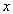i(ω): modal response: strain in terms of nodal value: stress in terms of nodal value